﻿ Four-fundamental forces, real mechanism

# Force withoug virtual particles.

Summary

## The current physics uses unreal force.

### [ Electric forces are caused by unreal virtual photons ? ]

(Fig.1)  Electric force is generated by emitting virtual photons ?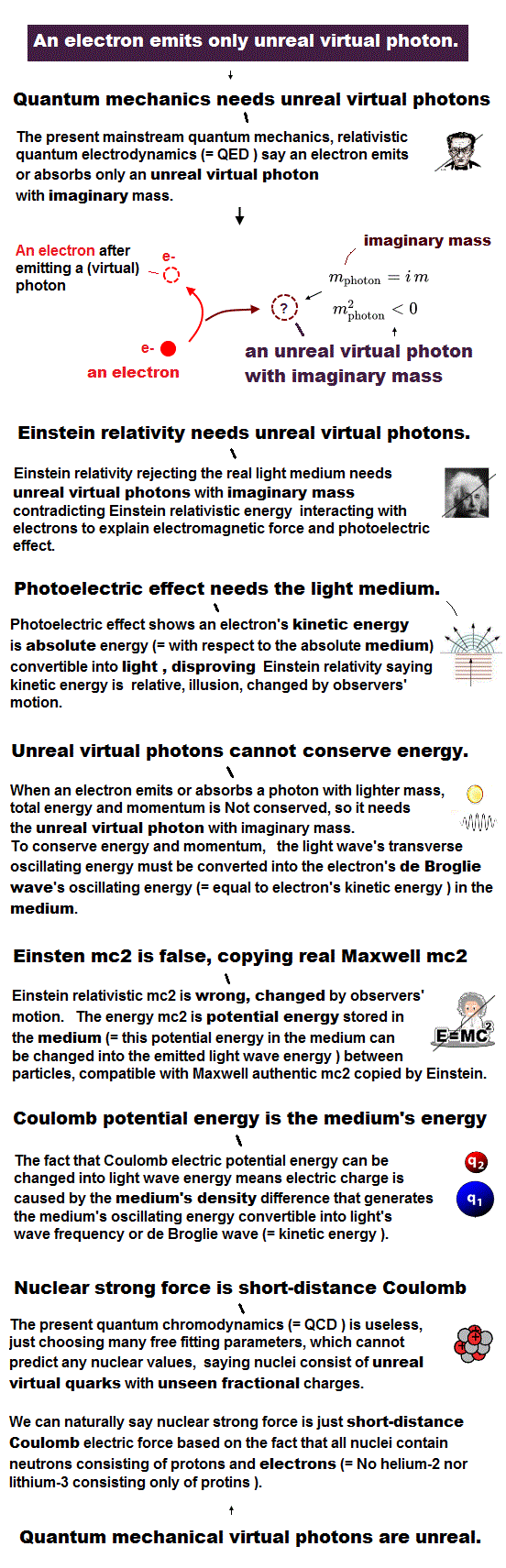The current quantum theory uses unreal virtual particles as force mediator and particle physics.

This virtual particles can have "imaginary mass (m2 < 0)", disobeying Einstein mc2, which cannot be observed as real particle ( this p.5, this p.6 ).

Forces such as electromagnetic are real, but they are caused by unreal virtual particles ?  This is why the current physics is wrong, and must be replaced by real one.

## Calculating virtual photon's abnormal mass.

### [ When an electron emits a photon, it becomes "virtual", NOT real ! ]

(Fig.2)  Total energy and momentum must be conserved.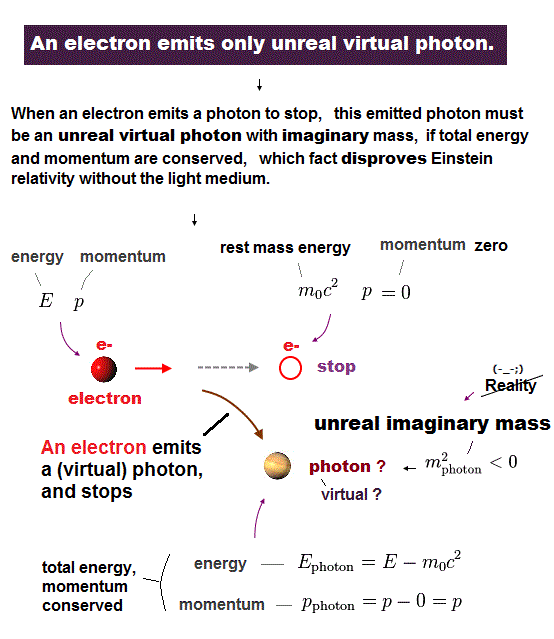When an electron emits a photon (= light particle ? ), this photon always must be virtual (= Not real ) with imaginary mass ( m2 < 0 ) !

In all reactions, total energy (= E ) and momentum (= p ) must be conserved.

The initial energy and momentum of an electron are E and p, respectively.  This electron stops after emitting a photon.

According to Einstein mass relation, the energy of the stationary electron is rest mass energy (= m0c2 ), and its momentum is zero.

Due to conservation law, the energy and momentum of the emitted photon is E - m0c2, and p, respectively.

## Einstein's energy and momentum relation.

### [ Particle's energy (= E ), momentum (= p), rest mass (= m0 ) ]

(Fig.3)  ↓ Einstein relativistic relation.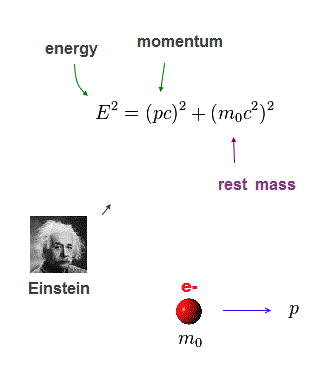Acccording to Einstein relativity, all ( real ) particles have to obey his energy-momentum-mass relation.

E, p and m0 are each particle's energy, momentum and rest mass, respectively.

## Emitted photon's mass squared is negative !

### [ Photon emitted from a electron has "imaginary" mass ( m2 < 0 ) ! ]

(Fig.4)  Photon with negative mass squared is virtual, NOT real.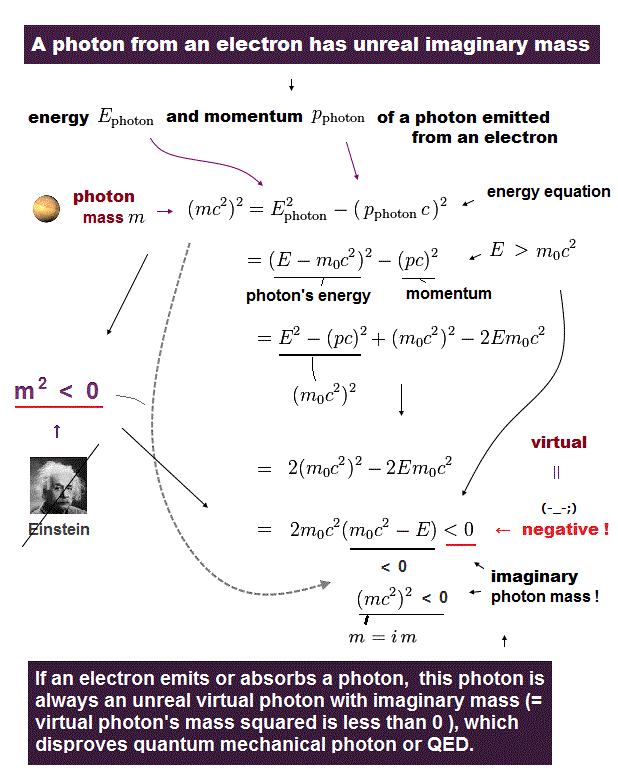Inserting the energy and momentum of the emitted photon into Einstein's relation, you'll easily find this emitted photon has unreal mass (= its mass squared is negative ! ).

In Fig.4, rest mass energy (= m0c2 ) is always smaller than total energy (= E = the sum of rest mass and kinetic energies ), so the final result is negative.

So when a photon emitted from an electron conserves total energy and momentum, its mass squared becomes negative (= imaginary mass ) and unreal.

It means a photon model in quantum field theory fails and we have to consider other realistic wave model involving the "whole atom" and medium.

## Fractional-charge quark lacks reality.

### [ We cannot separate or detect quark (gluon). ]

(Fig.5)  ↓ A neutron consists of a proton and an electron, Not quarks !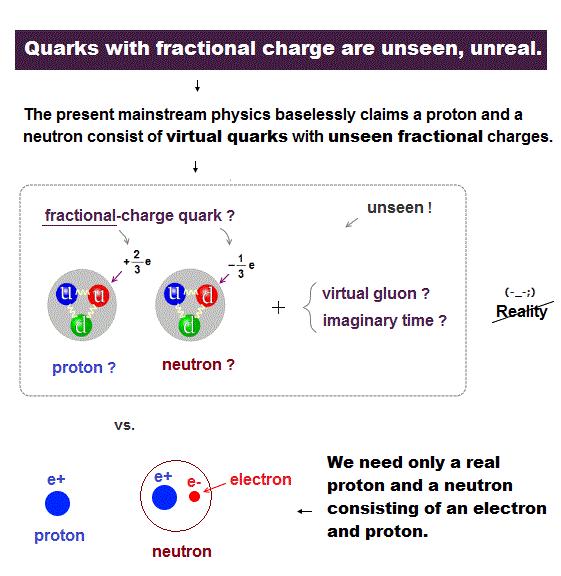The current physics says a proton and a neutron consist of fractional-charge quarks, which can Not be separated or detected.

They insist a nucleus is filled with unreal virtual sea quark-gluon, and governed by imaginary time and extradimension.

The fact of neutron decay tells us each neutron simply consists of a proton and an electron, Not unseen quarks !

The current nuclear physics lacks reality, full of imaginary concepts and artificial adjustable parameters ( this p.5 ), so useless and prevents nuclear fusion research.

## A neutron decays into a proton and an electron.

### [ Weak boson (= 80 times heavier than a proton !? ) is unreal. ]

(Fig.6)  ↓ The current physics uses unreal virtual W boson.A neutron decays into a proton and electron in beta decay.

The current physics insists imaginary quarks inside neutron decays into virtual Weak boson, which is 80 times heavier than a proton ( or a neutron ) !  ← lacking reality.

It is impossible that a neutron emits weak boson, which is much heavier than a neutron itself, so they call it unreal virtual particle.

We don't need these contradictory, unreal, useless particles or artificial models.
We can simply say a neutron decays into a proton and an electron !

## Short-distance Coulomb causes strong force.

### [ We don't need virtual fractional-charge quark or gluon ! ]

(Fig.7)  ↓ The current nuclear model is full of unreal concepts.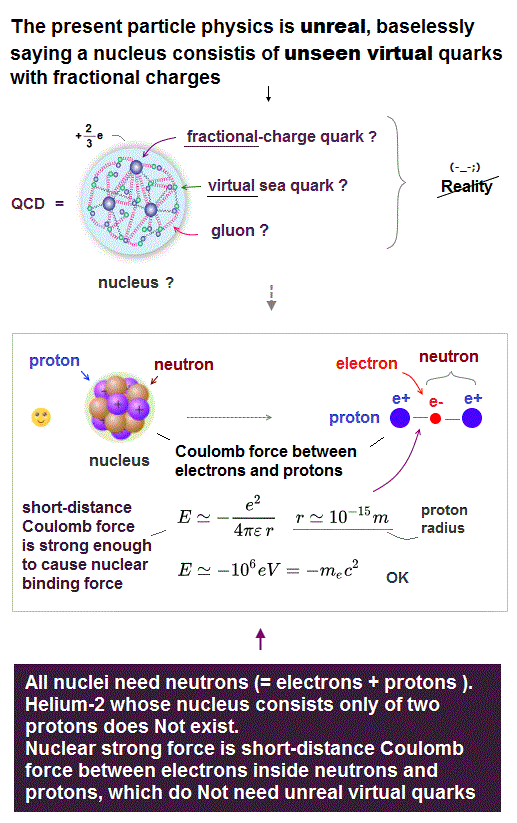The current nuclear model is full of imaginary concepts, such as unseen fractional-charge quark, virtual sea quark-gluon ..

We need to describe nuclei using realistic concepts !
Nuclei always consist of protons and neutrons.

As protons increase inside nuclei, neutrons increase, too.  A neutron consists of a proton and an electron.

The current physics completely ignores these electrons inside nuclei !
Electrons very close to protons must exert strong attractive force.

From the proton radius (= 10-15 m ), we can estimate Coulomb energy between an electron and a proton inside nuclei, which is very big.

This short-distance Coulomb energy becomes about 1 MeV (= 106 eV ), which is almost equal to electron's mass energy (= mc2 )

So, we can say strong nuclear force is caused by Coulomb force between a proton and a electron inside neutron, instead of imaginary quark- gluon.

According to Virial theorem, particle's kinetic energy is equal to minus total energy.  Electrons can probably approach protons until they are accelerated to light speed (= mc2 ).

## Electrons act as "adhesive" inside nuclei.

### [ Electrons strongly stick protons together at a short distance. ]

(Fig.8)  ↓ Helium-2 without electrons do Not exist.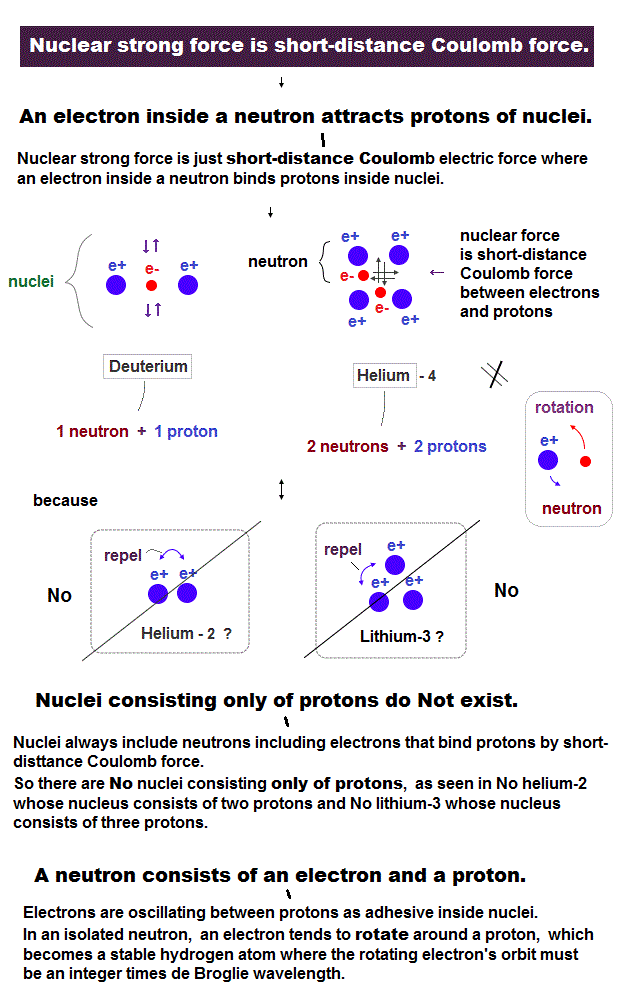In Helium isotope, there are only Helium-3 and Helium-4, both of which contain electrons (= neutron ).

Helium-2 consisting only of two protons without electrons cannot exist.

This fact proves that electrons inside nuclei act as "adhesive" among protons inside nuclei.

Nuclei include more protons than electrons, so electrons are oscillating among protons, instead of rotating around protons.

When there is only a pair of an electron and a proton like in neutron, the electron tends to rotate around the proton, become a hydrogen where the elecrton's orbital is stable, when its length is an integer times de Broglie wavelength.

## Why electromagnetic wave is transverse ?

### [ Electron's orbit changes → emit electromagnetic wave. ]

(Fig.9)  ↓ Electron emits light in the "transverse" direction.Electromagnetic wave (= light, photon ? ) is said to be "transverse" wave.

If the light is emitted from a single electron, it becomes unreal virtual photon with imaginary mass, when the total energy and momentum are conserved.

But the light = electromagnetic wave is a real thing !
We need to think about the whole system of not only an electron but also a nucleus.

Electron's de Broglie wave was experimentally confirmed, which inteference indicates the existence of some " medium".

An electron orbits around a nucleus, their total momentum of an electron and a nucleus is zero.

Even after an electron loses energy emitting light and its orbit shrinks, the sum of the electron's and nuclear momentums remains zero.

So an electromagnetic wave which is "pushed out" in the transverse direction carries mainly "energy" rather than "momentum".  This light wave occurs in the medium (= Not emitted from an electron ! ) around the atom.

If the light is emitted in the both directions, or the whole system of an electron and nucleus is pushed a little in the opposite direction of the emitted light, both the energy and momentum are conserved.

This is the mechanism of "real" electromagnetic transverse wave emission without virtual photon.

Basically, an electron loses kinetic energy ( and shrinks its orbital radius ), when the electron's de Broglie waves crashes into each other by destructive interference.

The direction (= parallel to electron moving direction ) in which an electron crushes into its destructive de Broglie wave corresponds to "light amplitude oscillating (= transverse ) direction" where an electron's kinetic energy is transfered to the medium (= around electron ) potential energy which becomes higher (= as strong as electron's kinetic energy ) by being compressed from both sides (= moving electron crushes its destructive de Broglie wave in the opposite directions ).

This "compressed medium accumulating potential energy from electron's lost kinetic energy" is pushed out as light wave in the direction (= longitudinal direction of light wave ) perpendicular to electron's original moving direction.

## Electromagnetic wave is "complex" wave.

### [ Light is a combination of "transverse" and "longitudinal" waves. ]

(Fig.10)  ↓ Elecromagnetic wave is similar to water wave.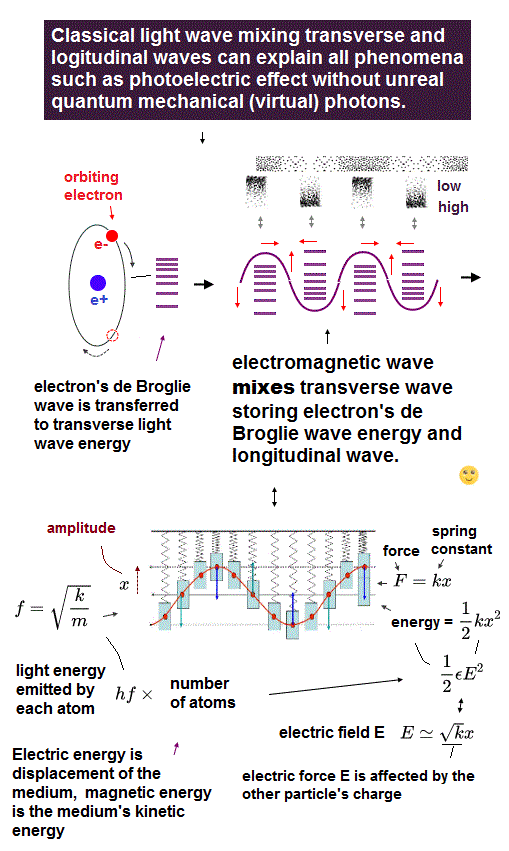Light is transverse wave, but it has momentum also in the longitudinal direction, so it is "complex" wave like water wave.

Correctly, the light wave is like a collection of longitudinal waves with different phases (= multiple layers of longitudinal waves ) in transverse direction, considering the fact that the light wave is emitted from electron's (circular periodic movement's) de Broglie wave (= after electron's de Broglie waves collide with each other and experience destructive interference. ).

Unlike the simple water or sound wave (= those waves not caused by electron's microscopic motion by Coulomb force but just by macroscopic overall mechanical vibration ), the light wave is emitted from the precise electron's periodic small motion around positive nucleus attracted by Coulomb force.

So the light wave is emitted and pushed out from destructive interference of electron's de Broglie wave as a collection of longitudinal waves with different phases in sync with the periodic electron's motion (so, in the transverse direction, different longitudinal light waves in line with periodic electron's movement are connected with each other by balancing the medium's pressures between low and high density areas caused by medium's oscillation in the upper figure rather than simple transverse oscillation ).

Transverse and longitudinal waves are caused by "medium osillation" in the vertical or horizontal directions.

"Complex" wave is a combination of these vertical and horizontal oscillations, local medium returns to its original position like spring pushed by its surrounding medium.

In the normal air or water, the "spring" strength (= to get the medium back to its original position ) in the transverse direction is too weak without gravity or tsunami.

If the light medium is denser, its transverse vibration of the light can affect electron's orbital energy, and its longitudinal vibration causes Complton scattering.

## Neutrino is "wave" like light.

### [ Neutrino is "longitudinal" wave with light speed c. ]

(Fig.11)  Why "neutrino" and "light" always travel at light-speed c ?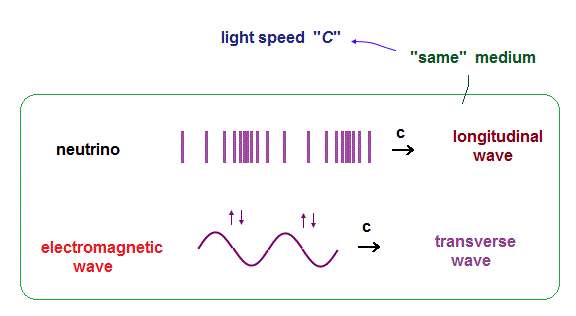It is known both neutrino and electromagnetic wave always travel at light-speed c.
"Constant" speed means these things are some oscillations travelling in the same common medium.

We can naturally think that this neutrino is longitudinal wave, the light is "transverse (= complex )" wave travelling through the common medium with the light speed.

If the neutrino is longitudinal wave, it doesn't interact with electron's orbit in the transverse direction, which can explain why "neutrino wave" can pass through things like ghost.

## True mechanism of photoelectric effect.

### [ Why is the emitted light energy equal to hf (= frequency ) ? ]

(Fig.12) light energy = h × frequency f.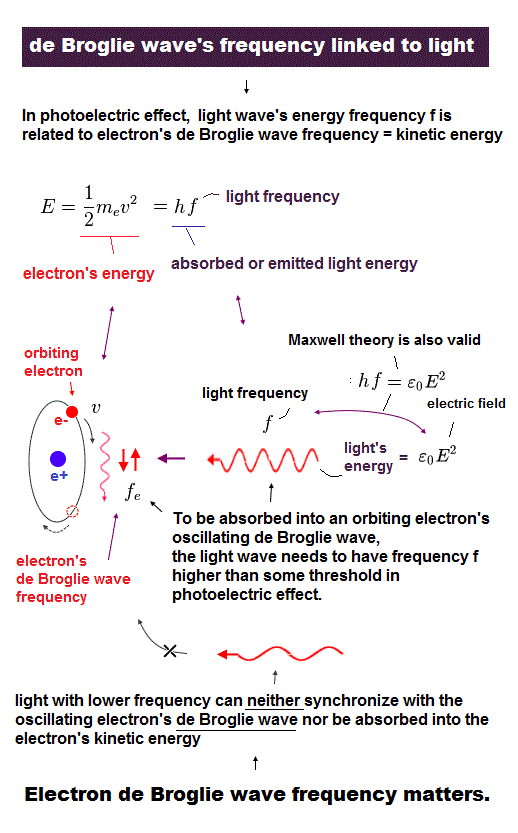Photoelectric effect shows the light emitted from atom has the energy of hf ( h is Planck constant, f is light frequency ).

We suppose an electron with kinetic energy of 1/2mev2 ( me and v are electron's mass and velocity. ) emits light.

( To be correct, the whole atom of electron and nucleus emits light, avoiding virtual photon, but the nuclear kinetic energy is too small, can be neglected ).

## Electron and light frequencies.

### [ Frequency f = velocity (= v ) divided by wavelength (= λ ) ]

(Fig.13)  de Broglie wavelength (= λ ) = h/p, p is momentum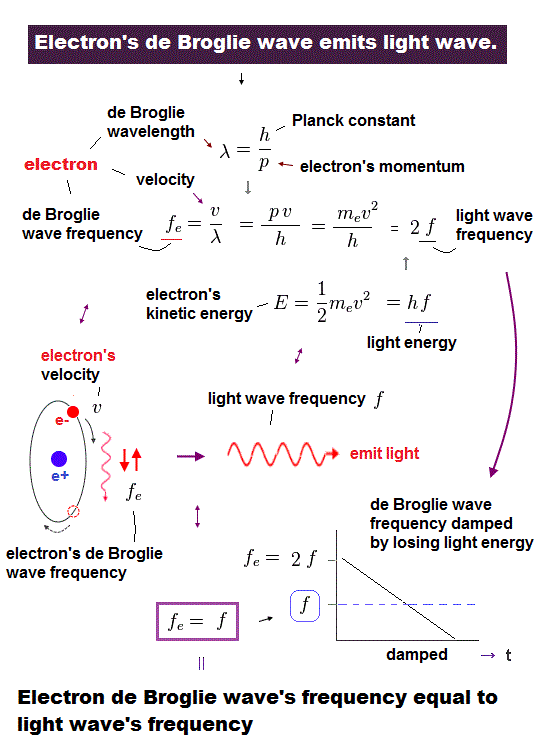According to wave relation, frequency f is equal to velocity (= v ) divided by wavelength ( λ ).

When an electron is moving at a velocity of v, its de Broglie wavelength becomes λ = h/mv = h/p ( p is electron's momentum ).

From Fig.12 and Fig.13, we get the relation of electron's de Broglie wave frequency (= fe ) equal to twice emitted light frequency (= 2f ).

## Average frequency of "damped" electron's oscillation is equal to emitted light (= f ).

(Fig.14) Oscillating electron emits light and gradually loses its energy.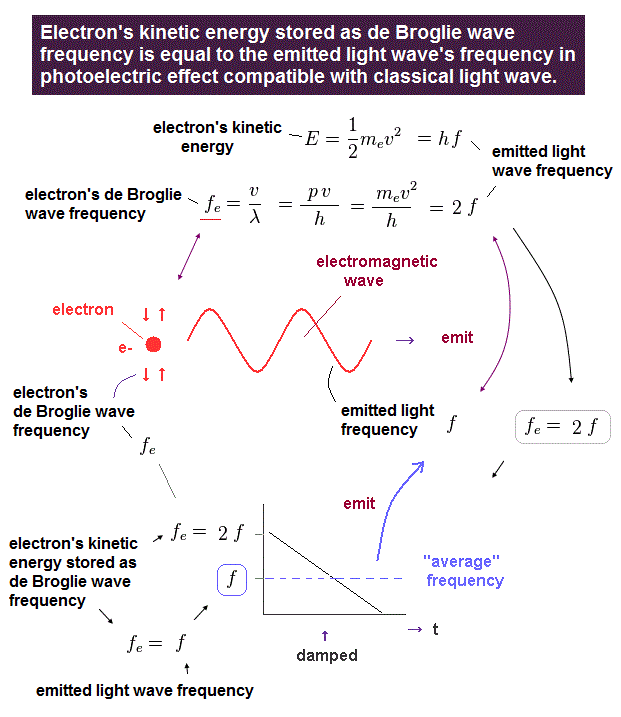We think about the case in which oscillating electron gradually emits light and loses its kinetic energy.
The initial electron's frequency is equivalent to 2 × f (= emitted light frequency ), as shown in Fig.13.

The more light the electron emits, it loses more energy, and its oscillation becomes slower ( to be zero ).
It means the average electron's frequency becomes half of the original value (= 1/2 × fe = f ) .

As a result, we can prove there is an important relation between electron's and emitted light frequencies.  Planck constant (= h ) connects light and electron.

This is the mechanism of photoelectric effect using "real" light and electron.

## Why proton and electron charges are exactly the same amount ?

### [ The current quantum mechanics cannot answer why the universe contains the same amount of ±charges ! ]

(Fig.15)  Imbalance in "field pressure" causes plus, minus charges.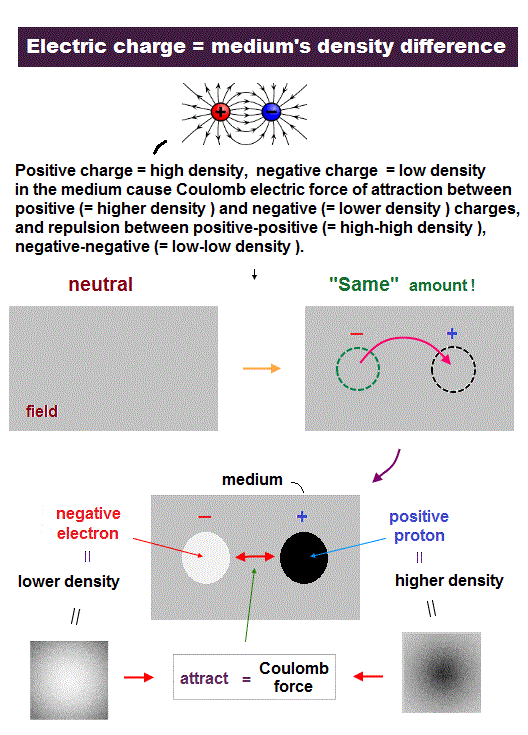Quantum mechanics cannot answer "why the universe contains exactly the same amount of plus, minus charges" as protons and electrons.  And they have NO definite answer, "why a proton is much heavier than an electron".

If we suppose Coulomb force is caused by the difference in field pressures (= density ), we can naturally answer all these basic questions.

In Fig.15 upper, some amount of field matters are transferred to another places.
This causes a pair of "thin" area with lower pressure, and "dense" area with "higher" pressure in the field.

If the field matters are unevenly distributed, they try to go back to the uniform density, which is the origin of Coulomb attraction.  "Dense" proton is naturally heavier than "thin" electron.

Due to pressure balance, the combinations of "dense-dense (= +, + )" and "thin-thin (= -, - )" areas repel each other, which is why the same charges repel each other in Coulomb forces.

Due to energy conservation, this density imbalance in medium can be kept, which is more realistic than unreal quantum virtual photon model.

## "Dense" proton is much heavier than "thin" electron.

### [ Total momentum conservation causes an light electron to move much faster. ]

(Fig.16)  An proton is 1840 times heavier (= denser ) than an electron.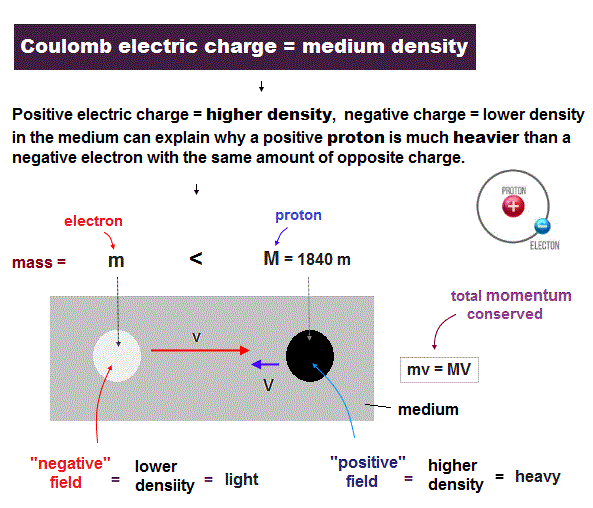It is known that a proton is about 1840 times heavier than a electron.  If we suppose, a proton (= positive field ) is 1840 times denser than an electron (= negative field ), this big difference in mass can be explained.

Due to imbalance in field densities (= "positive" is dense, "negative" is thin ), the opposite charges attract and start to move towards each other.

Total momentum ( and center of mass ) must be conserved due to the law of action and reaction.
So a "light" electron must move much ( 1840 × ) faster than a heavy proton during the same time.

## True mechanism of Lorentz magnetic force.

### [ Mechanical Coulomb force change = magnetic force ? ]

(Fig.17)  ↓ Parallel currents = attract, antiparallel = repel.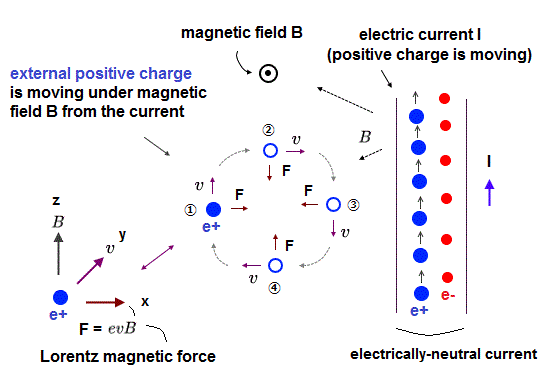In Lorentz magnetic force, when electric currents are in the same direction, they attract each other.  When the currents are opposite, they repel each other.  Why ?

In Fig.17 left, when an electron is moving in the same direction as electric current, Their Coulomb repulsion becomes weaker due to the target electrons moving away.

When the electron is moving in the opposite to the current, their Coulomb repulsion becomes stronger because the electrons are moving towards each other.

This indicates that Coulomb force travels through some "medium" at finite light speed c, and this force is affected by two charges' movement.2019/1/23 updated. Feel free to link to this site.